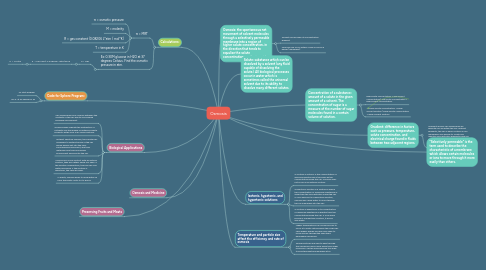# OsmosisCaroline Trew

Osmosis

Get Started. It's Free
or sign up with your email addressOsmosis## 2. Calculations:

### 2.1. π = MRT

2.1.1. π = osmotic pressure

2.1.2. M = molarity

2.1.3. R = gas constant (0.08206 L*atm / mol*K)

2.1.4. T = temperature in K

### 2.2. Ex: 0.30M glucose in H2O at 37 degrees Celsius. Find the osmotic pressure in atm.

2.2.1. π = MRT

2.2.1.1. π = 0.30 mol/L x 0.08206 L*atm/mol*K

2.2.1.1.1. π = 7.6 atm